2.1 原型

2.2 案例

2.3 解读

3.1 输入输出原型

3.2 输入解读

3.3 输出解读

## 第1章 RNN神经网络的理论基础

https://blog.csdn.net/HiWangWenBing/article/details/121387285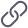https://blog.csdn.net/HiWangWenBing/article/details/121387285

## 第2章 torch.nn.RNN类

### 2.1 原型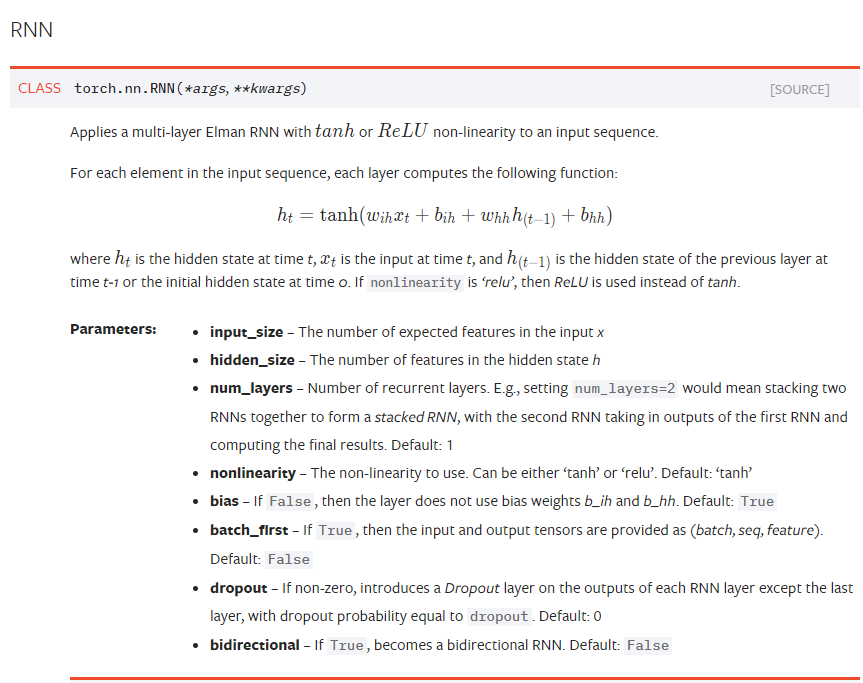### 2.2 案例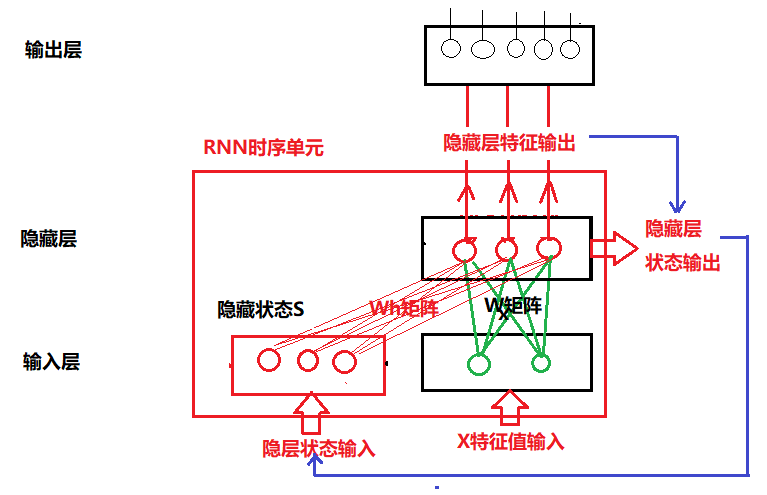### 2.3 解读

（1）input_size输入样本的向量长度

（2）hidden_size：“时序”单元中隐藏层的输出特征的向量长度

（3）num_layers: 隐藏层堆叠的层数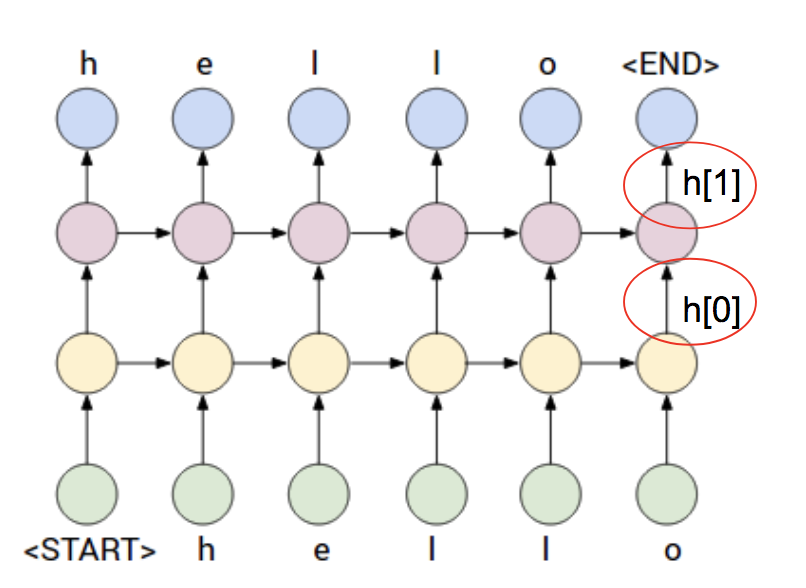在上图案例中，num_layers= 1。

（4）nonlinearity：定义激活函数

`nonlinearity == 'tanh'或'relu':`

（5）bias：是否需要偏置项

（6）batch_first：定义

```batch_first==True：               (batch, seq, feature)
batch_first==False（torch默认）： (seq, batch, feature)
```

torch之所以把seq放在最前面，这是因为在时序逻辑处理中，通常会优先批量读取多个序列数据。

（7）dropout: 是否需要dropout隐层的输出

（8）bidirectional：是否为双向RNN网络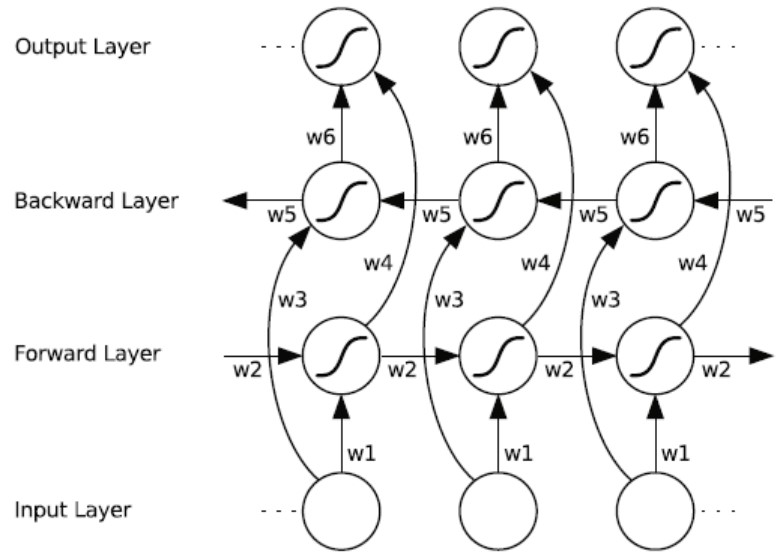双向网络中，两个方向上，各自有独立的权重矩阵和状态张量。

## 第3章 forward前向运算的输入与输出

### 3.1 输入输出原型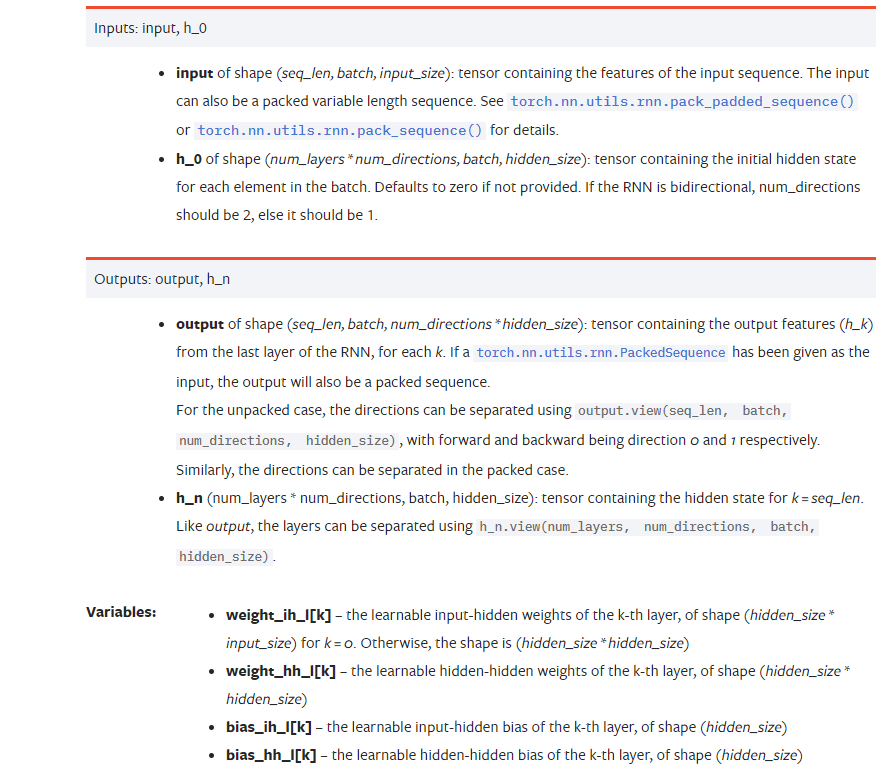### 3.2 输入解读

• input：用于存放输入样本的张量，张量的形状如下：

math:`(L, N, H_{in})` when ``batch_first=False`` or :

math:`(N, L, H_{in})` when ``batch_first=True`

```N：batch size， 一次可以送个一个batch的数据，batch size描述的可以同时并行输入的序列串的个数。
L：sequence length，连续多个输入样本，一次性送入RNN网络或foward函数中，RNN会依次输出sequence length批次的输出。sequence length可以串行输入序列的个数。
H_{in} ：input_size
H_{out} ：hidden_size
```
• h_o： 用于存放RNN初始的隐藏状态，通常为上一时刻预测时隐层状态的输出，如果没有上一时刻，这设置全0.

### 3.3 输出解读

• output：RNN网络的输出
• h_n：    RNN网络隐层的输出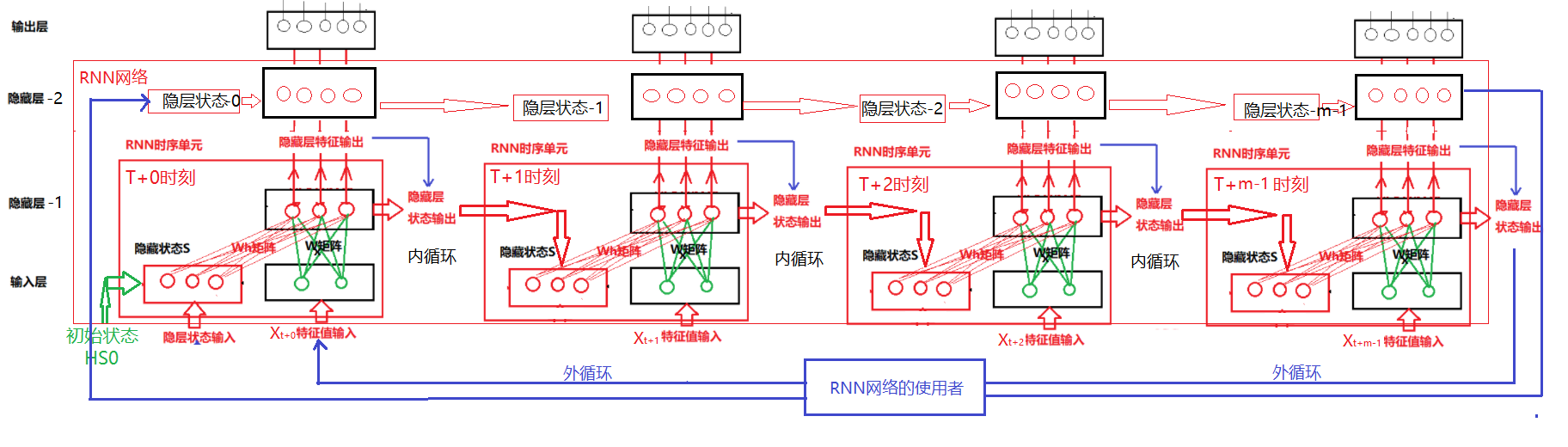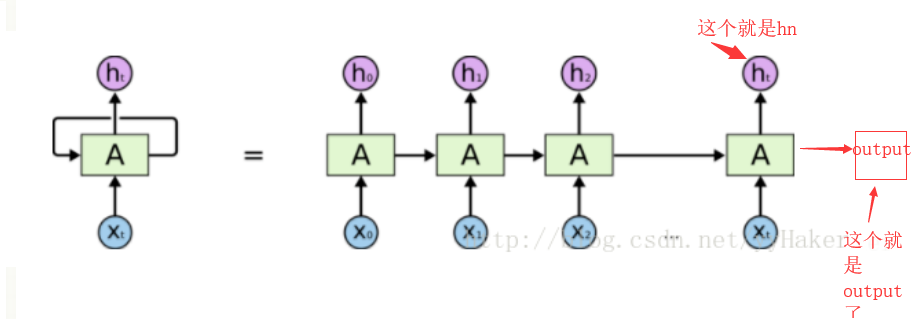hn就是RNN的最后一个隐含状态。

## 第4章 代码示例：Pytorch预定义RNN网络

（1）环境准备

``````#环境准备
import numpy as np              # numpy数组库
import math                     # 数学运算库
import matplotlib.pyplot as plt # 画图库
import time as time

import torch             # torch基础库
import torch.nn as nn    # torch神经网络库
import torch.nn.functional as F

import torchnlp
from torchnlp.word_to_vector import GloVe
# from torchnlp.word_to_vector import Glove

print("Hello World")
print(torch.__version__)
print(torch.cuda.is_available())
print(torch.version.cuda)
print(torch.backends.cudnn.version())``````
```Hello World
1.10.0
True
10.2
7605```

（2）定义一个序列化输入

``````# 定义输入序列的长度
seq_len = 3

#定义batch的长度
batch_size = 1

# input_size: 输入特征的数量/维
input_size  = 2

# 定义输入样本
input = torch.randn(seq_len, batch_size, input_size)
print(input.shape)
print(input)``````
```torch.Size([3, 1, 2])
tensor([[[-0.1100,  0.7480]],

[[-0.0672, -1.2424]],

[[ 0.1957,  0.4846]]])```

（3）定义RNN网络

``````# 定义RNN网络
# input_size: 输入特征的数量/维

# hidden_size（横向）：隐藏层的size（不是层数），即输出序列的长度，也是输入序列的长度
hidden_size = 3
# num_layers （纵向）： 隐藏层的堆叠层数
num_layers = 1

# 定义H0的状态
h0 = torch.zeros(num_layers, batch_size, hidden_size)
print(h0.shape)``````
`torch.Size([1, 1, 3])`

``````# 定义卷积神经网络
rnn = torch.nn.RNN(input_size= input_size, hidden_size = hidden_size, num_layers = num_layers, bias=True, bidirectional=False)
print(rnn)

#显示神经网络的参数
# 备注：
# 输入权重矩阵weight_hh_l0是 hidden_size * input_size ？ 不是说权重共享的吗？
# 隐层权重矩阵weight_hh_l0是 hidden_size * hidden_size ？

print(rnn.parameters)
for key, value in rnn.state_dict().items():
print(key)
print(value)``````
```RNN(2, 3)
<bound method Module.parameters of RNN(2, 3)>
weight_ih_l0
tensor([[-0.2329, -0.3762],
[-0.2569, -0.3311],
[-0.4779,  0.2690]])
weight_hh_l0
tensor([[ 0.1738, -0.4826,  0.2800],
[-0.5386, -0.0141, -0.2643],
[ 0.5265,  0.1451, -0.1797]])
bias_ih_l0
tensor([ 0.1088, -0.0520,  0.0557])
bias_hh_l0
tensor([-0.1980, -0.3961, -0.2472])```

ih：输入到隐藏层的权重矩阵=2 * 3， 这是因为这里定义的隐藏层的输入特征=2， 输出特征为3.

hh：隐藏状态到隐藏层的权重矩阵=3 * 3， 这是因为这里定义的隐藏层的状态特征=3， 输出特征为3，他们比如是相等的。

（4）网络输出：单输入，单输出

``````# 单个单词输入
# 0输出矩阵 = seq_len *  hidden_size，
# h输出矩阵 =    1    *  hidden_size
input_single = input
input_single = input_single.reshape(1, batch_size, input_size)

output, h = rnn(input_single, h0)

print("input：单输入")
print(input_single.shape)
print(input_single)

print("\noutput：单输出")
print(output.shape)
print(output)

# h是最后的输出
print("\nhiden:隐藏状态输出")
print(h.shape)
print(h)``````
```input：单输入
torch.Size([1, 1, 2])
tensor([[[0.0749, 0.5568]]])

output：单输出
torch.Size([1, 1, 3])

hiden:隐藏状态输出
torch.Size([1, 1, 3])

（4）网络输出：序列输入，序列输出

``````# 序列输入（多个单词组成序列）
# 0输出矩阵 = seq_len *  hidden_size，
# h输出矩阵 =    1    *  hidden_size
print("input：序列输入")
print(input.shape)
print(input)

output, h = rnn(input, h0)
print("\noutput：序列输出")
print(output.shape)
print(output)

# h是最后的输出
print("\nhiden:隐藏状态输出")
print(h.shape)
print(h)``````
```input：序列输入
torch.Size([3, 1, 2])
tensor([[[ 0.0749,  0.5568]],

[[ 0.2010, -0.1058]],

[[-1.7882,  1.2671]]])

output：序列输出
torch.Size([3, 1, 3])
tensor([[[-0.3060, -0.5728, -0.0773]],

[[ 0.1050, -0.2649, -0.4977]],

hiden:隐藏状态输出
torch.Size([1, 1, 3])
```

### python基础教程百度云-python从入门到精通视频百度云盘下载_编程大乐趣的博客-程序员秘密

python入门教程－9－Python编程风格(1).zippython入门教程－8－Python编程语言基础技术框架(4)之函数介绍.zippython入门教程－7－Python编程语言基础技术框架(3)之print输出.zippython入门教程－6－Python编程语言基础技术框架(2).zippython入门教程－60－Python执行环境及doctes.zippython入门教程－5－P...

### HDU3764(Cyclic Nacklace)_;w the endhdu_Splaying的博客-程序员秘密

Cyclic NacklaceProblem DescriptionCC always becomes very depressed at the end of this month, he has checked his credit card yesterday, without any surprise, there are only 99.9 yuan left. he is too distressed and thinking about how to tide over the last

### dockers镜像的制作_李氏程序员的博客-程序员秘密

[[email protected] ~]# docker imagesREPOSITORY TAG IMAGE ID CREATED SIZEcentos latest 75835a67d134 3 month...